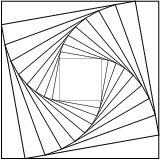# PyX — Example: drawing2/insert.py

0.4 KB
20.0 KB
2.2 KB
0.9 KB
2.1 KB

## Inserting transformed canvases into canvases```from math import sin, cos, radians
from pyx import *

angle = 10

cc = canvas.canvas()
cc.stroke(path.rect(-2, -2, 4, 4))

c = canvas.canvas()
for i in range(10):
c.insert(cc, [trafo.rotate(i*angle), trafo.scale(factor**i)])
c.writeEPSfile("insert")
c.writePDFfile("insert")
c.writeSVGfile("insert")
```

### Description

Similar to the drawing of paths onto canvases, it is also possible to insert one canvas into another. You may specify additional transformations which are applied to the canvas which is inserted.

The example code shows how a canvas `cc` containing a square can be inserted several times into another canvas `c`. The applied transformation first rotates the square around the origin and then performs a scaling. Note that not only the size of the square gets scaled, but everything that is drawn on the canvas, also the linewidth of the square.

In geometrical operations, it is often necessary to perform calculations. This is conveniently done by importing the mathematics capability of Python. In the example, we calculate the scaling factor from the rotation angle such that the corners of the rotated smaller square reside on the sides of the larger square.